# Create and use a box plot

Box plots provide a quick visual summary of the variability of values in a dataset. They show the median, upper and lower quartiles, minimum and maximum values, and any outliers in the dataset. Outliers can reveal mistakes or unusual occurrences in data. A box plot is created using a number or rate/ratio field on the y-axis.

Box plots can answer questions about your data, such as: How is my data distributed? Are there any outliers in the dataset? What are the variations in the spread of several series in the dataset?

## Examples

A market researcher is studying the performance of a retail chain. A box plot of the annual revenue at each store can be used to determine the distribution of sales, including the minimum, maximum, and median values.

The box plot above shows the median sales amount is \$1,111,378 (shown by hovering over the chart or using the Flip card button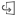to flip the card over). The distribution seems fairly even, with the median being in the middle of the box and the whiskers being a similar size. There are also low and high outliers, which gives the analyst an indication of which stores are over- and underperforming.

To delve deeper into the data, the analyst decides to create individual box plots for each region where the stores are located. She does this by changing the Group by field to Region. The result is four individual box plots that can be compared to discern information about each region.

Based on the box plots, the analyst can tell that there are few differences between regions; the medians are consistent across the four box plots, the boxes are similar sizes, and all regions have outliers at both the minimum and maximum ends. However, the whiskers for the Northern and Central regions are slightly more compact than the Bay Area and Southern regions, which implies that those regions have more consistent performance than the others. In the Bay Area and Southern regions, the whiskers are a bit longer, which implies those regions have stores that are performing poorly, as well as stores that are performing well. The analyst may want to focus her analysis on those two regions to find out why there is such a variation in performance.

## Create a box plot

To create a box plot, complete the following steps:

1. Select one of the following data options:
• A number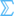or rate/ratio field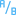.
• A numberor rate/ratio fieldplus a string field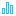.
##### Note:

You can search for fields using the search bar in the data pane.

2. Create the box plot using the following steps:
1. Drag the selected fields to a new card.
2. Hover over the Chart drop zone.
3. Drop the selected fields on Box Plot.
##### Tip:

You can also create charts using the Chart menu above the data pane or the Visualization type button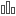on an existing card. For the Chart menu, only charts that are compatible with your data selection will be enabled. For the Visualization type menu, only compatible visualizations (including maps, charts, or tables) will be displayed.

##### Note:

Box plots created from database datasets must have at least five records. Box plots with fewer than five records are most likely to occur when grouping your box plot using a string field or applying a filter to your dataset or card. Database datasets are available through database connections in Insights in ArcGIS Enterprise and Insights desktop.

## Usage notes

The Layer options button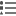opens the Layer options pane. The Layer options pane contains the following functions:

• The Legend tabis available when a group by field is applied to the x-axis of the chart. If a group by field is used, side-by-side box plots are created, with each box plot representing the spread of data in each category. The pop out legend button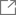displays the legend as a separate card on your page. You can use the legend to make selections on the chart. To change the color associated with a value, click the symbol and choose a color from the palette or enter a hex value.
• The Appearance tab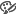changes the symbol color on the chart (single symbol only).

Use the Visualization type buttonto switch directly between a box plot and other visualizations, such as a graduated symbols map, summary table, or histogram. If the box plot includes a Group by field, the visualization can be changed to charts, such as a line graph or column chart.

Use the Flip card buttonto view the back of the card. The Card info tab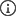provides information about the data on the card and the Export data tab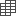allows users to export the data from the card.

A key feature for a box plot is the determination of outliers. Outliers are values that are much larger or smaller than the rest of the data. Whiskers on a box plot represent the threshold beyond which values are considered outliers. If there are no outliers, the whiskers will stretch to the minimum and maximum values in the dataset. In Insights, the range for the lower and upper outlier values are indicated on the box plot as circles linked by dotted lines.

Each statistic or range in the box plot can be selected by clicking the chart.

When you create a box plot, a result dataset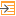with the input fields and output statistics will be added to the data pane. The result dataset can be used to find answers with nonspatial analysis using the Action button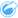.

## How box plots work

A box plot consists of the following components:

LabelComponentDescription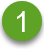Whisker

The range of data less than the first quartile and greater than the third quartile. Each whisker has 25 percent of the data. Whiskers typically cannot be more than 1.5 times IQR, which sets the threshold for outliers.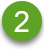Box

The range of data between the first and third quartiles. 50 percent of the data lies within this range. The range between the first and third quartile is also known as the Inter Quartile Range (IQR).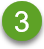Maximum

The largest value in the dataset or the largest value that is not outside the threshold set by the whiskers.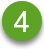Third quartile

The value where 75 percent of the data is less than the value, and 25 percent of the data is greater than the value.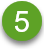Median

The middle number in the dataset. Half of the numbers are greater than the median and half are less than the median. The median can also be called the second quartile.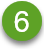First quartile

The value where 25 percent of the data is less than the value, and 75 percent of the data is greater than the value.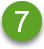Minimum

The smallest value in the dataset or the smallest value that is not outside the threshold set by the whiskers.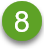Outliers

Data values that are higher or lower than the limits set by the whiskers.